## Solving Quadratics by Completing the Square

The expression x 2 + bx can be made into a square trinomial by adding to it a certain value. This value is found by performing two steps:

1. Multiply b (the coefficient of the “ x‐term”) by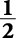.

2. Square the result.

##### Example 1

Find the value to add to x 2 + 8 x to make it become a square trinomial.

x 2 + 8 x

Multiply the coefficient of the “ x‐term” by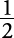.Square that result.

(4) 2 = 16

So 16 must be added to x 2 + 8 x to make it a square trinomial.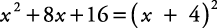Finding the value that makes a quadratic become a square trinomial is called completing the square. That square trinomial then can be solved easily by factoring.

##### Example 1

Solve the equation x 2 – 10 x = –16 by using the completing the square method.

x 2 – 10 x = –16

Multiply coefficient of “ x‐term” by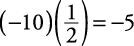Square the result.

(–5) 2 = 25

Add 25 to both sides of the equation.To solve quadratic equations by using the completing the square method, the coefficient of the squared term must be 1. If it isn't, then first divide both sides of the equation by that coefficient and then proceed as before.

##### Example 3

Solve 2 x 2 – 3 x + 4 = 0 by using the completing the square method.

2 x 2 – 3 x + 4 = 0

Get the coefficient of the squared term to be 1.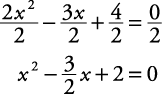Isolate the variable terms.Complete the square.Use the square root property.Back to Top
A18ACD436D5A3997E3DA2573E3FD792A